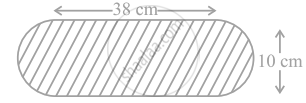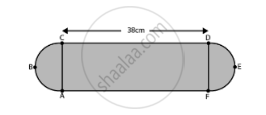# Find the area of the flower bed (with semi-circular ends) shown in figure. - Mathematics

Sum

Find the area of the flower bed (with semi-circular ends) shown in figure.#### Solution

Length and breadth of the rectangular portion AFDC of the flower bed are 38 cm and 10 cm.

Area of the flower bed = Area of the rectangular portion + Area of the two semicircles.Area of rectangle AFDC = Length × Breadth

= 38 × 10

= 380 cm2

Both ends of flower bed are semi-circle in shape.

Diameter of the semi-circle = Breadth of the rectangle AFDC = 10 cm

Radius of the semi-circle = 10/2 = 5 cm

Area of the semi-circle = (pir^2)/2

= 25(pi/2)  cm^2

As, there are two semi-circles in the flower bed,

Area of two semi-circles = 2 xx ((pir^2)/2)

= 25 pi  cm^2

Therefore, total area of flower bed = (380 + 25pi)  cm^2.

Concept: Area of Circle
Is there an error in this question or solution?

#### APPEARS IN

NCERT Mathematics Exemplar Class 10
Chapter 11 Area Related To Circles
Exercise 11.3 | Q 6 | Page 126

Share